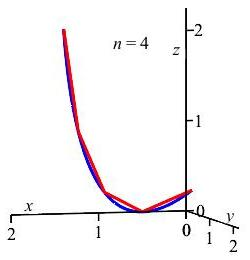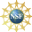# 8. Properties of Curves

## 1. Arc Length of a Curve

To find a formula for the length of a parametric curve, $$\vec{r}(t)$$, from $$A=\vec{r}(a)$$ to $$B=\vec{r}(b)$$, we approximate the curve by straight line segments and sum the lengths of the segments. In the limit, as we use more segments which are shorter and shorter, this turns into an integral for the exact length of the curve.

We start by dividing the curve, $$\vec r(t)=(x(t),y(t))$$, into $$n$$ segments by partitioning the $$t$$ values at $$t_0,t_1,\cdots,t_{i-1},t_{i},\cdots,t_{n}$$. As a shorthand, we define: $x_i=x(t_i), \qquad y_i=y(t_i), \qquad z_i=z(t_i)$ We use the distance formula in three dimensions to determine the length of each of the straight line segments from $$\vec{r}\left(t_{i-1}\right)$$ to $$\vec{r}\left(t_{i}\right)$$. Finally, we sum these straight line differences from $$i=1$$ to $$i=n$$ to get an approximation to the length of the curve: $L\approx\sum_{i=1}^n \sqrt{\left(x_i-x_{i-1}\right)^2+ \left(y_i-y_{i-1}\right)^2+\left(z_i-z_{i-1}\right)^2}$ In the plot, a curve, shown in blue, is partitioned into $$4$$ approximating line segments, in red. The length of the blue curve is approximated by summing the lengths of the red line segments.Next we note that $$x_i-x_{i-1}=\Delta x=\dfrac{\Delta x}{\Delta t}\Delta t$$. The same holds true for the $$y$$ and $$z$$ differences. Substituting these into the previous equation, we get \begin{aligned} L&\approx\sum_{i=1}^n \sqrt{\Delta x^2+\Delta y^2+\Delta z^2} \\ &\approx\sum_{i=1}^n \sqrt{ \left(\dfrac{\Delta x}{\Delta t}\Delta t\right)^2 +\left(\dfrac{\Delta y}{\Delta t}\Delta t\right)^2 +\left(\dfrac{\Delta z}{\Delta t}\Delta t\right)^2} \\ &\approx\sum_{i=1}^n \sqrt{ \left(\dfrac{\Delta x}{\Delta t}\right)^2 +\left(\dfrac{\Delta y}{\Delta t}\right)^2 +\left(\dfrac{\Delta z}{\Delta t}\right)^2}\,\Delta t \end{aligned}

This approximation becomes exact in the limit as the number of segments $$n$$ becomes infinite and $$\Delta t$$ goes to $$0$$, as shown in the animation. At the same time the quotient $$\dfrac{\Delta x}{\Delta t}$$ approaches the derivative $$\dfrac{dx}{dt}$$. Thus, the limit of the sum becomes the integral: \begin{aligned} L&=\lim_{\Delta t\longrightarrow 0} \sum_{i=1}^n \sqrt{ \left(\dfrac{\Delta x}{\Delta t}\right)^2 +\left(\dfrac{\Delta y}{\Delta t}\right)^2 +\left(\dfrac{\Delta z}{\Delta t}\right)^2}\,\Delta t \\ &=\int_a^b \sqrt{\left(\dfrac{dx}{dt}\right)^2 +\left(\dfrac{dy}{dt}\right)^2 +\left(\dfrac{dz}{dt}\right)^2}\,dt \end{aligned}

Notice that the square root in this formula is just the length of the velocity. So $L=\int_a^b |\vec{v}|\,dt$

### Arc Length Differential and Formulas for the Arc Length

The (Scalar) Differential of Arc Length is the differential: \begin{aligned} ds&=\sqrt{dx^2+dy^2+dz^2} \\ &=\sqrt{\left(\dfrac{dx}{dt}\right)^2 +\left(\dfrac{dy}{dt}\right)^2 +\left(\dfrac{dz}{dt}\right)^2}\,dt=|\vec{v}|\,dt \end{aligned} If a curve runs between the points $$A$$ and $$B$$, then the Arc Length of the Curve is the integral: $L=\int_A^B\,ds=\int_A^B \sqrt{dx^2+dy^2+dz^2}$ where the endpoints $$A$$ and $$B$$ are the limits on the integral. If we specify a parametrization (since there could be more than one way to parametrize the curve) as $$\vec{r}(t)$$, then the endpoints are $$A=\vec{r}(a)$$ and $$B=\vec{r}(b)$$ and we write: $L=\int_a^b |\vec{v}|\,dt$ where the limits on the integral are the values of $$t$$ at $$A$$ and $$B$$.

Compute the arclength of the twisted cubic $$\vec{r}(t)=\left(t,t^2,\dfrac{2}{3}t^3\right)$$ between $$t=0$$ and $$t=3$$.

The velocity is $$\vec{v}=(1,2t,2t^2)$$ and its length is $|\vec{v}|=\sqrt{1+4t^2+4t^{4}} =\sqrt{(1+2t^2)^2}=1+2t^2.$ So its arc length is \begin{aligned} L&=\int_{(0,0,0)}^{(3,9,18)}ds =\int_0^3 |\vec{v}|\,dt =\int_0^3 (1+2t^2)\,dt \\ &=\left[t+\dfrac{2}{3}t^3\right]_0^3=3+18=21 \end{aligned}

Compute the circumference of the circle $$\vec{r}(\theta)=(R\cos(\theta),R\sin(\theta),3)$$.

$$L=2\pi R$$
Notice this is the formula you already know for computing the circumference of a circle -- in fact, this is one way that formula is derived!

The circumference of a circle is the arc length of $$1$$ revolution, from $$\theta=0$$ to $$\theta=2\pi$$. So the circumference is $L=\int_0^{2\pi} |\vec{v}|\,d\theta$ To compute this, we need the magnitude of the velocity vector, which is \begin{aligned} |\vec{v}| &=\sqrt{\left(\dfrac{dx}{d\theta}\right)^2 +\left(\dfrac{dy}{d\theta}\right)^2 +\left(\dfrac{dz}{d\theta}\right)^2} \\ &=\sqrt{(-R\sin\theta)^2+(R\cos\theta)^2+0^2} \\ &=\sqrt{R^2(\sin^2\theta+\cos^2\theta)}=R \end{aligned} So the circumference is \begin{aligned} L=\int_0^{2\pi} |\vec{v}|\,d\theta =\int_0^{2\pi} R\,d\theta =2\pi R \end{aligned}

Notice this is the formula you already know for computing the circumference of a circle -- in fact, this is one way that formula is derived!

If the parameter happens to be $$z$$, while $$x$$ and $$y$$ are functions of $$z$$:   $$x=x(z)$$ and $$y=y(z)$$,   then the arc length differential becomes $ds=\sqrt{\left(\dfrac{dx}{dz}\right)^2+\left(\dfrac{dy}{dz}\right)^2+1}\,dz$ and the arc length integral is: $L=\int_a^b\,ds=\int_a^b \sqrt{\left(\dfrac{dx}{dz}\right)^2+\left(\dfrac{dy}{dz}\right)^2+1}\,dz$ where $$a$$ and $$b$$ are now the values of $$z$$ at the endpoints. Similar formulas hold when the parameter is $$x$$ or $$y$$.

Compute the arclength of the helix   $$x=4\cos\left(\dfrac{1}{3}z\right), y=4\sin\left(\dfrac{1}{3}z\right)$$   between $$z=0$$ and $$z=18\pi$$.

$$L=30\pi$$

The arc length is \begin{aligned} L&=\int_a^b \sqrt{\left(\dfrac{dx}{dz}\right)^2 +\left(\dfrac{dy}{dz}\right)^2+1}\,dz \\ &=\int_0^{18\pi} \sqrt{\dfrac{16}{9}\sin^2\left(\dfrac{1}{3}z\right) +\dfrac{16}{9}\cos^2\left(\dfrac{1}{3}z\right)+1}dz \\ &=\int_0^{18\pi} \sqrt{\dfrac{16}{9}+1}dz =\left[\dfrac{5}{3}z\right]_0^{18\pi}=30\pi \end{aligned}

Notice this is the same as the helix parametrized as $$\vec{r}(\theta)=(4\cos\theta,4\sin\theta,3\theta)$$ for $$0 \le \theta \le 6\pi$$.

You can also practice computing the arc length of curves using the following two Maplets (requires Maple on the computer where this is executed):

Supported in part by NSF Grant #1123255